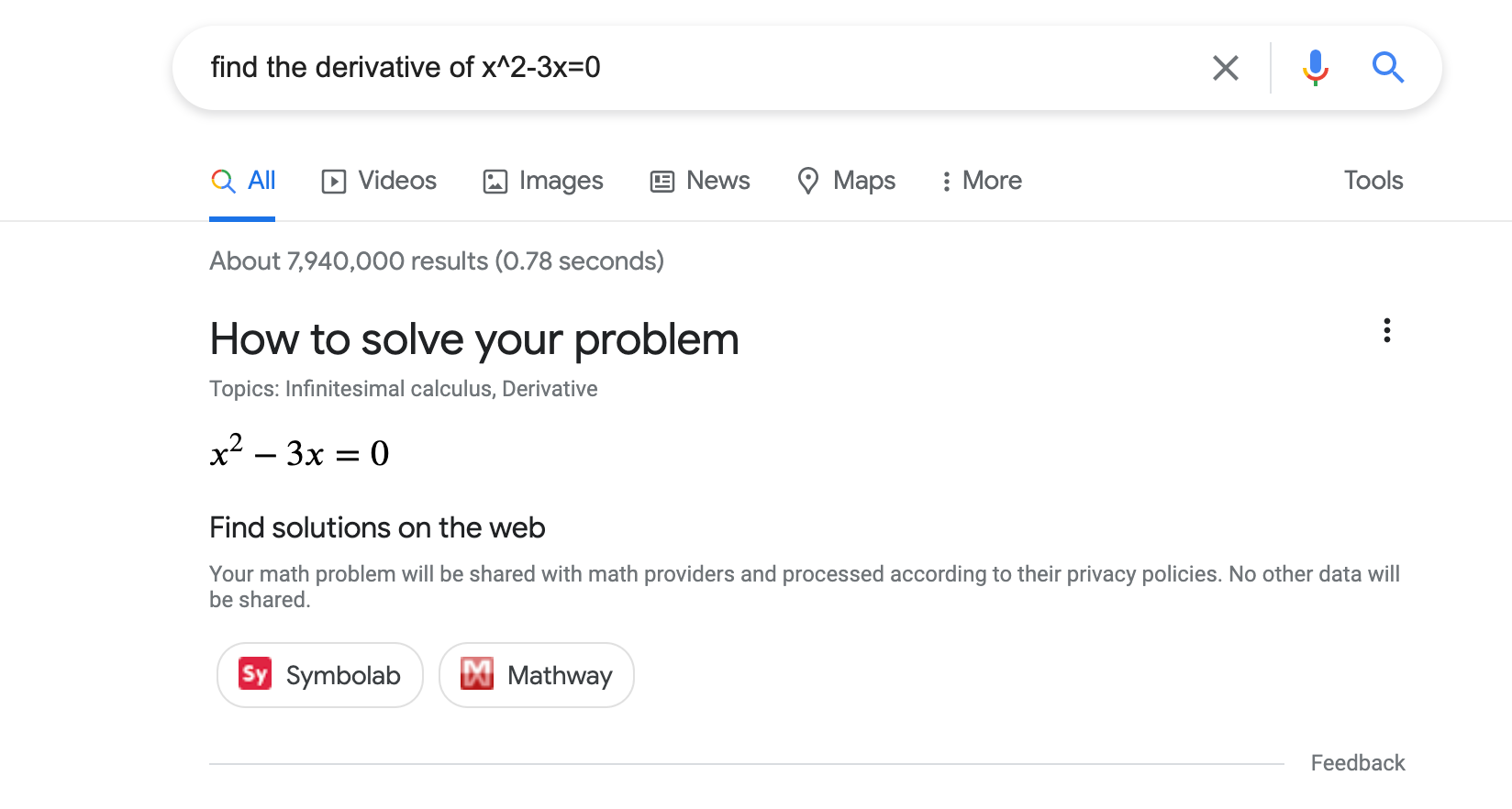# 数学求解器 (MathSolver) 结构化数据## 如何添加结构化数据

1. 添加必要属性。根据您使用的格式，了解在网页上的什么位置插入结构化数据
2. 遵循指南
3. 使用富媒体搜索结果测试验证您的代码，并修复所有严重错误。此外，您还可以考虑修正该工具中可能会标记的任何非严重问题，因为这些这样有助于提升结构化数据的质量（不过，要使内容能够显示为富媒体搜索结果，并非必须这么做）。
4. 部署一些包含您的结构化数据的网页，然后使用网址检查工具测试 Google 看到的网页样貌。请确保您的网页可供 Google 访问，不会因 robots.txt 文件、noindex 标记或登录要求而被屏蔽。如果网页看起来没有问题，您可以请求 Google 重新抓取您的网址
5. 为了让 Google 随时了解日后发生的更改，我们建议您提交站点地图Search Console Sitemap API 可以帮助您自动执行此操作。

## 示例

### 一项求解器操作

<html>
<title>An awesome math solver</title>
<body>
<script type="application/ld+json">
[
{
"@context": "https://schema.org",
"@type": ["MathSolver", "LearningResource"],
"name": "An awesome math solver",
"url": "https://www.mathdomain.com/",
"usageInfo": "https://www.mathdomain.com/privacy",
"inLanguage": "en",
"potentialAction": [{
"@type": "SolveMathAction",
"target": "https://mathdomain.com/solve?q={math_expression_string}",
"mathExpression-input": "required name=math_expression_string",
"eduQuestionType": ["Polynomial Equation","Derivative"]
}],
"learningResourceType": "Math solver"
},
{
"@context": "https://schema.org",
"@type": ["MathSolver", "LearningResource"],
"name": "Un solucionador de matemáticas increíble",
"url": "https://es.mathdomain.com/",
"usageInfo": "https://es.mathdomain.com/privacy",
"inLanguage": "es",
"potentialAction": [{
"@type": "SolveMathAction",
"target": "https://es.mathdomain.com/solve?q={math_expression_string}",
"mathExpression-input": "required name=math_expression_string",
"eduQuestionType": ["Polynomial Equation","Derivative"]
}],
"learningResourceType": "Math solver"
}
]
</script>
</body>
</html>

### 两项求解器操作

<html>
<title>An awesome math solver</title>
<body>
<script type="application/ld+json">
{
"@context": "https://schema.org",
"@type": ["MathSolver", "LearningResource"],
"name": "An awesome math solver",
"url": "https://www.mathdomain.com/",
"usageInfo": "https://www.mathdomain.com/privacy",
"inLanguage": "en",
"potentialAction": [{
"@type": "SolveMathAction",
"target": "https://mathdomain.com/solve?q={math_expression_string}",
"mathExpression-input": "required name=math_expression_string",
"eduQuestionType": "Polynomial Equation"
},
{
"@type": "SolveMathAction",
"target": "https://mathdomain.com/trig?q={math_expression_string}",
"mathExpression-input": "required name=math_expression_string",
"eduQuestionType": "Trigonometric Equation"
}],
"learningResourceType": "Math solver"
}
</script>
</body>
</html>

## 指南

### 技术指南

• MathSolver 结构化数据添加到您网站的首页。
• 确保您的主机负载设置能够承受频繁抓取
• 如果您在不同网址下托管了同一数学求解器的多个相同副本，请在网页的每个副本中使用规范网址

### 内容指南

• 我们不允许将宣传内容伪装成数学求解器，例如由第三方（如联属营销计划）发布的内容。
• 通过此功能呈现数学求解器时，您将对其准确性和质量负责。在质量审核流程中，如果我们发现一定数量的不准确数据，则根据不准确的程度，可能会从该功能中移除您的求解器，直到问题得到解决为止。适用范围：
• 求解器能够求解的数学题类型的准确性。
• 求解器声明可以求解的数学题的解法准确性。

## 结构化数据类型定义

### MathSolver

MathSolver 是一款工具，可以为学生、老师和其他人列出求解步骤，从而帮助他们求解数学题。请在网站的首页上使用 MathSolver 结构化数据。

potentialAction

SolveMathAction


{
"@type": "MathSolver",
"potentialAction": [{
"@type": "SolveMathAction",
"target": "https://mathdomain.com/solve?q={math_expression_string}",
"mathExpression-input": "required name=math_expression_string",
"eduQuestionType": "Polynomial Equation"
}]
}

potentialAction.mathExpression-input

Text

Google 会发送以下两种格式之一的 math_expression_string

• 
(math_expression)'
• 
d/dvariable math_expression

• (x^2+x)'
• d/dx (x^2+x)
• d/dy y^2+y

Google 会发送以下两种格式之一的 math_expression_string

• 
\int math_expression
• 
\int_{from}^{to} math_expression

• \int x^2+x
• \int_{0}^{2} x^2+x

Google 会发送以下两种格式之一的 math_expression_string

• 
\lim math_expression
• 
\lim_{variable\rightarrowvalue} math_expression

• \lim_{x\rightarrow0} sin(x)/x
• \lim_{y\rightarrow\infty} sin(y)/y
• \lim sin(x)/x
url

URL

MathSolver 的网址。

usageInfo

URL


{
"@type": "MathSolver",
"usageInfo": "https://www.mathdomain.com/privacy"
}
potentialAction.target

EntryPoint


{
"@type": "MathSolver",
"potentialAction": [{
"@type": "SolveMathAction",
"target": "https://mathdomain.com/solve?q={math_expression_string}"
}]
}

inLanguage

Text


{
"@type": "MathSolver",
"inLanguage": "es"
}
assesses


{
"@type": "MathSolver",
"assesses": "Polynomial Equation"
}
potentialAction.eduQuestionType


{
"@type": "SolveMathAction",
"eduQuestionType": "Polynomial Equation"
}


### LearningResource

LearningResource 表明标记对象是可帮助学生、老师和其他人开展教育学习的资源。请在网站的首页上使用 LearningResource

learningResourceType

Text


{
"@type": ["MathSolver", "LearningResource"],
"learningResourceType": "Math Solver"
}

## 数学题类型定义

MathSolver 附有提供特定数学题讲解的 HowTo 时，请将以下数学题类型列表用作 MathSolver.potentialActioneduQuestionTypeMathSolverassesses 字段。

Absolute Value Equation

Algebra

Arc Length

Arithmetic

Biquadratic Equation

Calculus

Characteristic Polynomial

Circle

Derivative

Differential Equation

Distance

Eigenvalue

Eigenvector

Ellipse

Exponential Equation

Function

Function Composition

Geometry

Hyperbola

Inflection Point

Integral

Intercept

Limit

Line Equation

Linear Algebra

Linear Equation

Linear Inequality

Logarithmic Equation

Logarithmic Inequality

Matrix

Midpoint

Parabola

Parallel

Perpendicular

Polynomial Equation

Polynomial Expression

Polynomial Inequality

Quadratic Equation

Quadratic Expression

Quadratic Inequality

Radical Equation

Radical Inequality

Rational Equation

Rational Expression

Rational Inequality

Slope

Statistics

System of Equations

Trigonometry

[{ "type": "thumb-down", "id": "missingTheInformationINeed", "label":"没有我需要的信息" },{ "type": "thumb-down", "id": "tooComplicatedTooManySteps", "label":"太复杂/步骤太多" },{ "type": "thumb-down", "id": "outOfDate", "label":"内容需要更新" },{ "type": "thumb-down", "id": "translationIssue", "label":"翻译问题" },{ "type": "thumb-down", "id": "samplesCodeIssue", "label":"示例/代码问题" },{ "type": "thumb-down", "id": "otherDown", "label":"其他" }]
[{ "type": "thumb-up", "id": "easyToUnderstand", "label":"易于理解" },{ "type": "thumb-up", "id": "solvedMyProblem", "label":"解决了我的问题" },{ "type": "thumb-up", "id": "otherUp", "label":"其他" }]